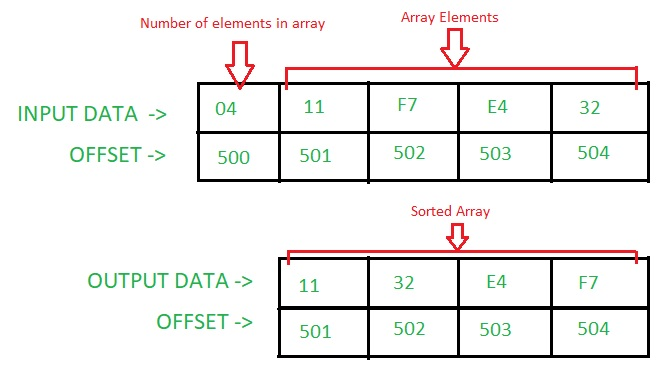# 8086 program for selection sort

Problem – Write an assembly language program in 8086 microprocessor to sort a given array of n numbers using Selection Sort.

Assumptions – The number of elements in the array is stored at offset 500. The array starts from offset 501.

Example –Algorithm –

1. We first find the smallest number in the array.
2. Swap the smallest number from the first element of the array.
3. Keep repeating the process till all elements are traversed.

Program –

Offset Mnemonics Comment
400 MOV DI, 501 DI < – 501
403 MOV SI, 500 SI < – 500
406 MOV CL, [SI] CL < – [SI]
408 XOR CH, CH CH < – CH ^(XOR) CH
40A INC SI SI < – SI+0001
40B DEC CX CX < – CX-0001
40C MOV BX, SI BX < – SI
40E MOV AH, CL AH < – CL
410 INC AH AH < – AH+01
412 MOV AL, [SI] AL < – [SI]
414 INC SI SI < – SI+0001
415 DEC AH AH < – AH-01
417 CMP AL, [SI] AL-[SI]
419 JC 41F If Carry Flag = 1, goto offset 41F
41B MOV AL, [SI] AL < – [SI]
41D MOV BX, SI BX < – SI
41F INC SI SI < – SI+0001
420 DEC AH AH < – AH-01
422 JNZ 417 If Zero Flag = 0, goto offset 417
424 MOV DL, [BX] DL < – [BX]
426 XCHG DL, [DI] DL < – > [DI]
428 XCHG DL, [BX] DL < – &gt [BX]
42A INC DI DI < – DI+0001
42B MOV SI, DI SI < – DI
42D LOOP 40C CX < – CX-0001; If Zero Flag = 0, goto offset 40C.
42F HLT End of program.

Explanation – Registers AH, AL, BX, CX, DL, SI, DI are used for general purpose:

```AL - Stored the smallest number
AH - Stores the counter for the inner loop
BX - Stores the offset of the smallest
number of each iteration of the outer loop
CX - Stores the counter for the outer loop
DL - Helps in swapping the elements
SI - Pointer
DI - Pointer ```
1. MOV SI, 500: stores 0500 in SI.
2. MOV CL, [SI]: stores the content at offset SI in CL.
3. XOR CH, CH: stores the result of logical operation XOR b/w CH and CH in CH.
4. INC SI: increase the value of SI by 1.
5. DEC CX: decrease the value of CX by 1.
6. MOV AH, CL: stores the contents of CL in AH.
7. CMP AL, [SI]: compares the content of AL with content at offset SI. If AL < [SI] – Sets Carry Flag(i.e. Carry Flag = 1).
8. JC 41F: jumps to offset 041F, if carry flag is set(1).
9. JNZ 417: jumps to offset 0417, if zero flag is reset(0).
10. XCHG DL, [BX]: swaps the content of DL with content at offset BX.
11. LOOP 40C: decrease the value of CX by 1 and check whether Zero Flag is set(1) or not. If Zero Flag is reset(0), then it jumps to offset 040C.
12. HLT: terminates the program.

My Personal Notes arrow_drop_upCheck out this Author's contributed articles.

If you like GeeksforGeeks and would like to contribute, you can also write an article using contribute.geeksforgeeks.org or mail your article to contribute@geeksforgeeks.org. See your article appearing on the GeeksforGeeks main page and help other Geeks.

Please Improve this article if you find anything incorrect by clicking on the "Improve Article" button below.